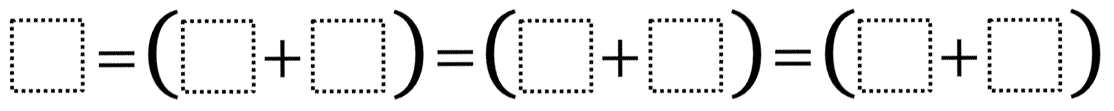Directions: Using the digits 1 to 9, at most one time each, make a true statement.### Hint

What has to be true about the pairs of numbers inside the parenthesis?

8 = (6+2) = (7+1) = (5+3)
9 = (8+1) = (7+2) = (6+3)
7 = (6+1) = (3+4) = (5+2)

Source: Owen Kaplinsky

### Check Also

Directions: Use the digits 1-9, at most once, to complete the equation. What is the …

1.Catherine Hashimoto

Can’t wait to have my students work on this!!!

2.9= (8+1) =(5+4) =(7+2)=(6+3)

3.4.9=(7+2)=(4+5)=(8+1)